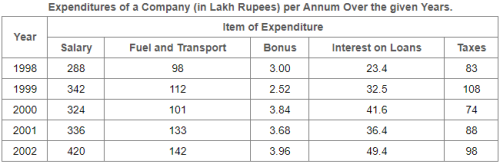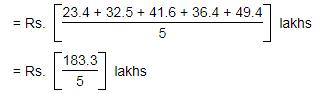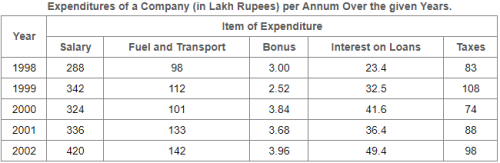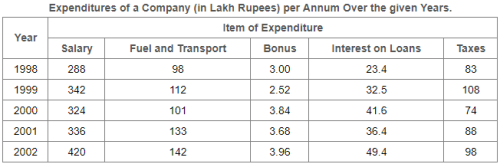Courses

# Test: Table Charts- 3

## 5 Questions MCQ Test UPSC Prelims Paper 2 CSAT - Quant, Verbal & Decision Making | Test: Table Charts- 3

Description
This mock test of Test: Table Charts- 3 for LR helps you for every LR entrance exam. This contains 5 Multiple Choice Questions for LR Test: Table Charts- 3 (mcq) to study with solutions a complete question bank. The solved questions answers in this Test: Table Charts- 3 quiz give you a good mix of easy questions and tough questions. LR students definitely take this Test: Table Charts- 3 exercise for a better result in the exam. You can find other Test: Table Charts- 3 extra questions, long questions & short questions for LR on EduRev as well by searching above.
QUESTION: 1

### Study the following table and answer the questions based on it.What is the average amount of interest per year which the company had to pay during this period?

Solution:

Average amount of interest paid by the Company during the given period= Rs. 36.66 lakhs.

QUESTION: 2

Solution:
QUESTION: 3

### Study the following table and answer the questions based on it.Total expenditure on all these items in 1998 was approximately what percent of the total expenditure in 2002?

Solution:
QUESTION: 4

Study the following table and answer the questions based on it.The total expenditure of the company over these items during the year 2000 is?

Solution:
QUESTION: 5

Study the following table and answer the questions based on it.The ratio between the total expenditure on Taxes for all the years and the total expenditure on Fuel and Transport for all the years respectively is approximately?

Solution: